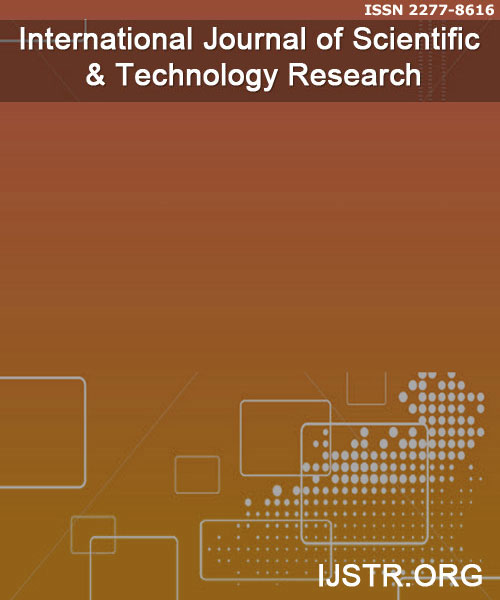## International Journal of Scientific & Technology Research

0.2
2019CiteScore

10th percentile
Powered byScopus coverage:
Nov 2018 to May 2020

 CALL FOR PAPERS
 AUTHORS
 DOWNLOADS
 CONTACT

# IJSTR >> Volume 5 - Issue 5, May 2016 EditionInternational Journal of Scientific & Technology Research Website: http://www.ijstr.org ISSN 2277-8616

 Approximate Solution For Time-Space Fractional Soil Moisture Diffusion Equation And Its Application [Full Text]   AUTHOR(S) Bhausaheb R. Sontakke, Veena V. Sangvikar (V. P. Kshirsagar)   KEYWORDS Time-Space fractional, soil moisture diffusion equation, finite difference, fractional derivatives, stability analysis, convergence analysis, Mathematica.   ABSTRACT The purpose of this paper is to develop an implicit finite difference method for time-space fractional soil moisture diffusion equation (TSFSMDE). We prove a detailed analysis of the scheme and generate the discrete model. Also, we prove the scheme is unconditionally stable and convergent. As an application of the scheme we solve some test problems and their solutions are represented graphically by powerful software Mathematica.   REFERENCES  Daniel Hillel, “Introduction to Soil Physics, Academic Press,” 1982.  Don Kirkham and W.L. Powers,”Advanced Soil Physics,” Wiley-Interscience, 1971.  D. B. Dhaigude and K. C. Takale,”A Weighted Average Finite Difference Scheme for One-Dimensional Penne’s Bioheat Equation”, Neural, Parallel and Scientific Computations, USA, 2010.  R. Goreno and F. Mainardi,”Approximation of Levy-Feller Diffusion by Random Walk,” Journal for Analysis and its Applications (ZAA) 18:231- 246, 1999.  R. Hilfer,”Applications of Fractional Calculus in Physics,” World Scientific, Singapore 2000.  M. Ilic, F. Liu, I. Turner and V. Anh, “Numerical approximation of a fractional-in-space diffusion equation,” I. Fractional Calculus and Applied Analysis 8, No 3:323-341,2005.  S. M. Jogdand, K. C. Takale and V. C. Borkar,”Fractional Order Finite Difference Scheme For Soil Moisture Diffusion Equation and its Applications,” IOSR Journal of Mathematics (IOSR-JM), Volume 5, 2013.  S. M. Jogdand, K. C. Takale and A. S. Jagtap, “Finite Difference Approximation for Space Fractional Soil Moisture Diffusion Equation and Its Application,” IOSR Journal of Mathematics (IOSR-JM) , Volume 8, 2013.  Y. Lin, C. Xu,”Finite difference/spectral approximations for the time-fractional diffusion equation,” J. Comput. Phys. 225 , pp.1533-1552:2007  F.Liu, P. Zhuang, V. Anh, I, Turner,”A Fractional Order Implicit Difference Approximation for the Space-Time Fractional Diffusion equation,” ANZIAM J.47 (EMAC2005), pp. C48-C68:2006.  M.M. Meerschaert, C. Tadjeran,”Finite difference approximations for two sided space-fractional partial differential equations,” Appl. Numer. Math. 56:80-90, 2006  M.M. Meerschaert, C. Tadjeran,”Finite difference approximations for fractional advection-dispersion flow equations,” J. Comput. Appl. Math. 172:65- 77, 2004.  Momani S, Odibat Z,”Numerical comparison of methods for solving linear differential equations of fractional order,” Chaos Soliton Fract 31:12481255, 2007.  Momani S, Odibat Z, “Numerical approach to differential equations of fractional order,” J Comp Appl Math 207(1):96110, 2007.  Pater A.C., Raats and Martinus TH. Ven Genuchten, “Milestones in Soil Physics,” J. Soil Science 171,1 2006.  I. Podlubny, “Fractional Differential equations,” Academic Press, San Diago, 1999.  M. Rehman, R. A. Khan, “A numerical method for solving boundary value problems for fractional differential equations,” Appl. Math. Model, 36(3), 894- 907, 2012.  B.Ross (Ed), “Fractional Calculus and Its Applications, Lecture Notes in Mathematics,” Vol.457, Springer-Verlag, New York, 1975.  S. Shen, F.Liu, “Error Analysis of an explicit Finite Difference Approximation for the Space Fractional Diffusion equation with insulated ends,” ANZIAM J.46 (E), pp. C871 - C887: 2005.  K. C. Takale, “Fractional Order Finite Difference Scheme for Space Fractional Boussinesq’s Equation and Its Applications,” Int Jr. of Mathematical Sciences and Applications, Vol.3, 2013.  K. C. Takale, D. B. Dhaigude and V. R. Nikam, “Douglas Higher Order Finite Difference Scheme for One-Dimensional Penne’s Bioheat Equation,” International Journal of Advanced Engineering and Applications, 2011.  K. C. Takale, V. R. Nikam and A. S. Shinde, ”Mittag-Leffleer Functions, its Computations and Applications to Differential Equations of Fractional Order,” Mathematical Modeling and Applied Soft Computing, Vol. 1,2012.  K. C. Takale, V. R. Nikam and A. S. Shinde,”Finite Difference Scheme for Space Fractional Diffusion Equation with Mixed Boundary Conditions,” American Jr. of Mathematics and Sciences, Vol. 2, 2013.  W. Wyss and W. R. Schneider,”Fractional Diffusion and wave equations,” J. Math. Phys. 30,1989.